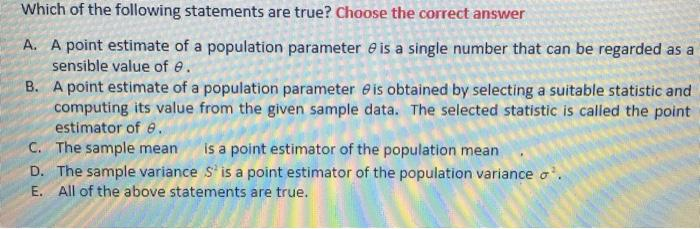# Question Which of the following statements are true? Choose the correct answer A. A point estimate of a population parameter e is a single number that can be regarded as a sensible value of e. B. A point estimate of a population parameter e is obtained by selecting a suitable statistic and computing its value from the given sample data. The selected statistic is called the point estimator of e. C. The sample mean is a point estimator of the population mean D. The sample variance S is a point estimator of the population variance o E. All of the above statements are true.G8LYXK The Asker · Probability and StatisticsTranscribed Image Text: Which of the following statements are true? Choose the correct answer A. A point estimate of a population parameter e is a single number that can be regarded as a sensible value of e. B. A point estimate of a population parameter e is obtained by selecting a suitable statistic and computing its value from the given sample data. The selected statistic is called the point estimator of e. C. The sample mean is a point estimator of the population mean D. The sample variance S is a point estimator of the population variance o E. All of the above statements are true.
More
Transcribed Image Text: Which of the following statements are true? Choose the correct answer A. A point estimate of a population parameter e is a single number that can be regarded as a sensible value of e. B. A point estimate of a population parameter e is obtained by selecting a suitable statistic and computing its value from the given sample data. The selected statistic is called the point estimator of e. C. The sample mean is a point estimator of the population mean D. The sample variance S is a point estimator of the population variance o E. All of the above statements are true.